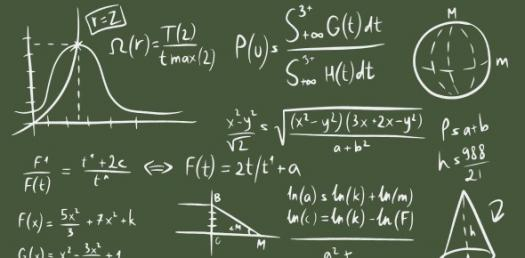# A Mathematical Exam About Ellipse & Hyperbola. Be A Mathematician

8 Questions | Attempts: 175SettingsA hyperbola is an open curve with two branches, the intersection of a plane with both halves of a double cone. The plane does not have to be parallel to the axis of the cone; the hyperbola will be symmetrical in any case. In mathematics, an ellipse is a plane curve surrounding two focal points, such that for all points on the curve, the sum of the two distances to the focal points is a constant. Share with quiz with your math classmates.

• 1.
It is the set of points in plane such that the difference of its distances from two fixed points is constant
• A.

Circle

• B.

Parabola

• C.

Ellipse

• D.

Hyperbola

• 2.
A segment passing through the focus of the ellipse/hyperbola that is perpendicular to the major axis.
• A.

Asymptote

• B.

Directix

• C.

Latus rectum

• D.

Transverse axis

• 3.
The segment made by the endpoints of the vertices of a hyperbola is called
• A.

Conjugate axis

• B.

Major axis

• C.

Minor axis

• D.

Transverse axis

• 4.
Is [e = .98 an ellipse] true or false?
• A.

True

• B.

False

• 5.
Is [Ellipse the best structure to follow in making a Cooling Tower in powerplant because of its efficiency in heat removing process ?] true or false?
• A.

True

• B.

False

• 6.
The standard equation 9x2 - 4y2 = 36  is
• A.

Option 1

• B.

Option 2

• C.

Option 3

• D.

Option 4

• 7.
What
• A.

Option 1

• B.

Option 2

• C.

Option 3

• D.

Option 4

• 8.
What is area of an ellipse?

## Related TopicsBack to top
×

Wait!
Here's an interesting quiz for you.